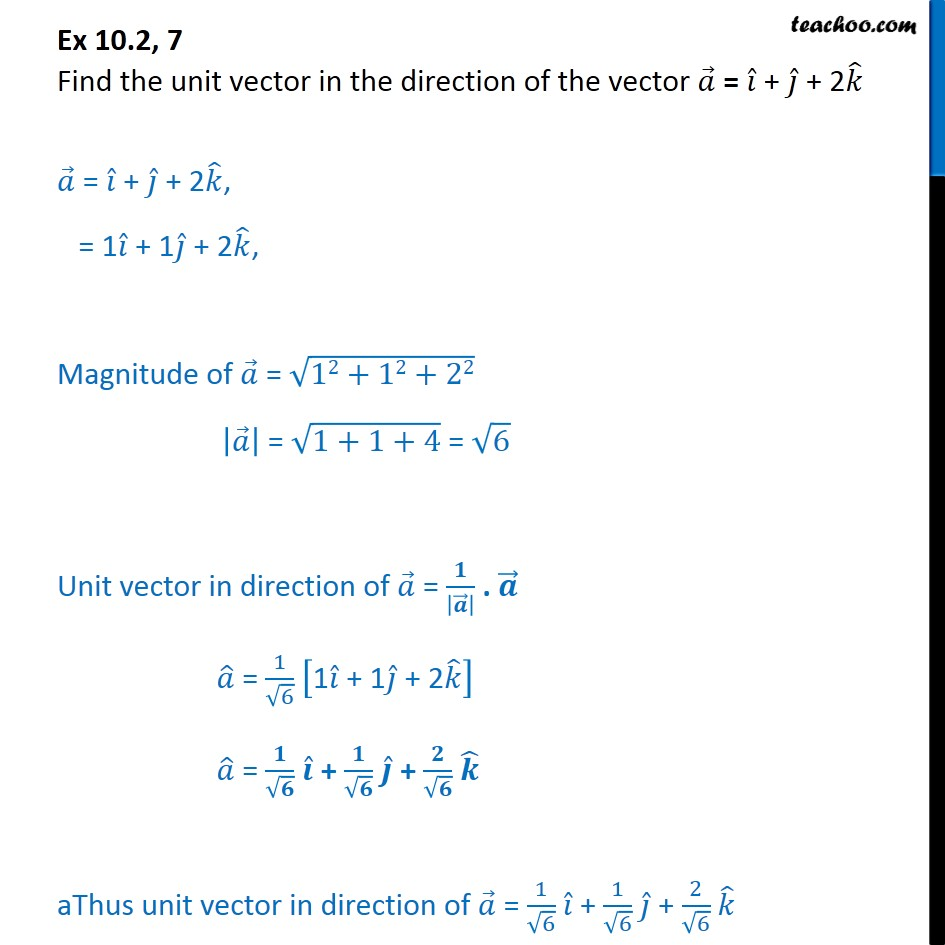1. Class 12
2. Important Questions for exams Class 12
3. Chapter 10 Class 12 Vector Algebra

Transcript

Ex 10.2, 7 Find the unit vector in the direction of the vector = + + 2 = + + 2 , = 1 + 1 + 2 , Magnitude of = 12+12+22 = 1+1+4 = 6 Unit vector in direction of = . = 1 6 1 + 1 + 2 = + + aThus unit vector in direction of = 1 6 + 1 6 + 2 6

Chapter 10 Class 12 Vector Algebra

Class 12
Important Questions for exams Class 12